Search by Topic

Resources tagged with Rotations similar to Complex Rotations:

Filter by: Content type:
Age range:
Challenge level:

There are 28 results

Broad Topics > Transformations and constructions > RotationsFootprints

Age 16 to 18 Challenge Level:

Make a footprint pattern using only reflections.Napoleon's Theorem

Age 14 to 18 Challenge Level:

Triangle ABC has equilateral triangles drawn on its edges. Points P, Q and R are the centres of the equilateral triangles. What can you prove about the triangle PQR?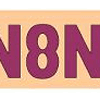Symmetric Trace

Age 14 to 16 Challenge Level:

Points off a rolling wheel make traces. What makes those traces have symmetry?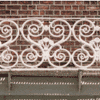Frieze Patterns in Cast Iron

Age 11 to 16

A gallery of beautiful photos of cast ironwork friezes in Australia with a mathematical discussion of the classification of frieze patterns.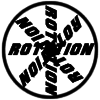Rots and Refs

Age 16 to 18 Challenge Level:

Follow hints using a little coordinate geometry, plane geometry and trig to see how matrices are used to work on transformations of the plane.A Roll of Patterned Paper

Age 14 to 16 Challenge Level:

A design is repeated endlessly along a line - rather like a stream of paper coming off a roll. Make a strip that matches itself after rotation, or after reflection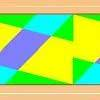Rotations Are Not Single Round Here

Age 14 to 16 Challenge Level:

I noticed this about streamers that have rotation symmetry : if there was one centre of rotation there always seems to be a second centre that also worked. Can you find a design that has only. . . .Attractive Tablecloths

Age 14 to 16 Challenge Level:

Charlie likes tablecloths that use as many colours as possible, but insists that his tablecloths have some symmetry. Can you work out how many colours he needs for different tablecloth designs?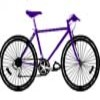Illusion

Age 11 to 16 Challenge Level:

A security camera, taking pictures each half a second, films a cyclist going by. In the film, the cyclist appears to go forward while the wheels appear to go backwards. Why?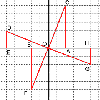The Matrix

Age 16 to 18 Challenge Level:

Investigate the transfomations of the plane given by the 2 by 2 matrices with entries taking all combinations of values 0. -1 and +1.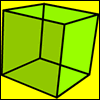Cubic Rotations

Age 14 to 16 Challenge Level:

There are thirteen axes of rotational symmetry of a unit cube. Describe them all. What is the average length of the parts of the axes of symmetry which lie inside the cube?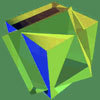Interpenetrating Solids

Age 16 to 18 Challenge Level:

This problem provides training in visualisation and representation of 3D shapes. You will need to imagine rotating cubes, squashing cubes and even superimposing cubes!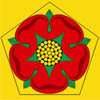Rose

Age 16 to 18 Challenge Level:

What groups of transformations map a regular pentagon to itself?The Frieze Tree

Age 11 to 16

Patterns that repeat in a line are strangely interesting. How many types are there and how do you tell one type from another?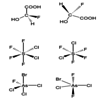Stereoisomers

Age 16 to 18 Challenge Level:

Put your visualisation skills to the test by seeing which of these molecules can be rotated onto each other.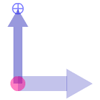Twizzle Arithmetic

Age 14 to 16 Challenge Level:

Arrow arithmetic, but with a twist.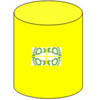Paint Rollers for Frieze Patterns.

Age 11 to 16

Proofs that there are only seven frieze patterns involve complicated group theory. The symmetries of a cylinder provide an easier approach.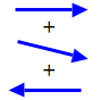Arrow Arithmetic 1

Age 14 to 16 Challenge Level:

The first part of an investigation into how to represent numbers using geometric transformations that ultimately leads us to discover numbers not on the number line.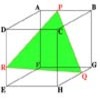Cut Cube

Age 16 to 18 Challenge Level:

Find the shape and symmetries of the two pieces of this cut cube.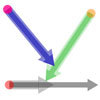Arrow Arithmetic 2

Age 14 to 16 Challenge Level:

Introduces the idea of a twizzle to represent number and asks how one can use this representation to add and subtract geometrically.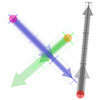Arrow Arithmetic 3

Age 14 to 16 Challenge Level:

How can you use twizzles to multiply and divide?Cubic Spin

Age 16 to 18 Challenge Level:

Prove that the graph of f(x) = x^3 - 6x^2 +9x +1 has rotational symmetry. Do graphs of all cubics have rotational symmetry?Get Cross

Age 14 to 16 Challenge Level:

A white cross is placed symmetrically in a red disc with the central square of side length sqrt 2 and the arms of the cross of length 1 unit. What is the area of the disc still showing?Coke Machine

Age 14 to 16 Challenge Level:

The coke machine in college takes 50 pence pieces. It also takes a certain foreign coin of traditional design...Robotic Rotations

Age 11 to 16 Challenge Level:

How did the the rotation robot make these patterns?In a Spin

Age 14 to 16 Challenge Level:

What is the volume of the solid formed by rotating this right angled triangle about the hypotenuse?Middle Man

Age 16 to 18 Challenge Level:

Mark a point P inside a closed curve. Is it always possible to find two points that lie on the curve, such that P is the mid point of the line joining these two points?Hand Swap

Age 14 to 16 Challenge Level:

My train left London between 6 a.m. and 7 a.m. and arrived in Paris between 9 a.m. and 10 a.m. At the start and end of the journey the hands on my watch were in exactly the same positions but the. . . .